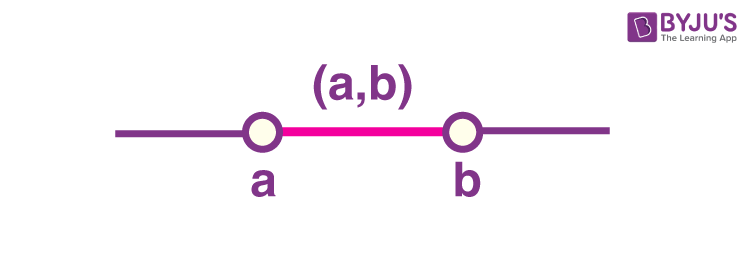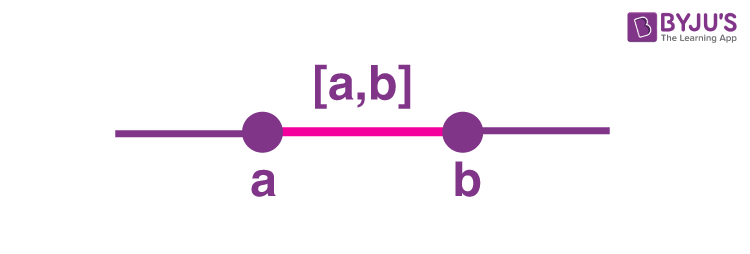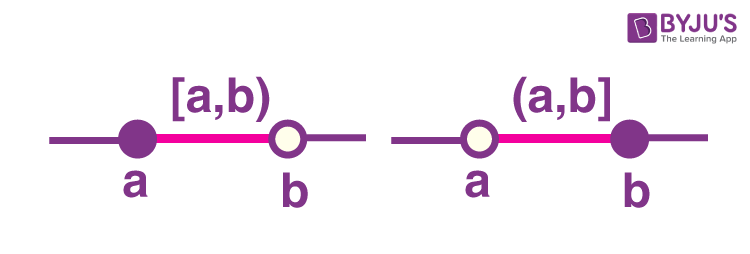# Intervals as Subsets of R

In mathematics, an interval can be defined as a set of real numbers that contains all real numbers lying within any two specific numbers of the set R. We already know about the subsets of set of real numbers and how to represent them. From the subsets of real numbers, we can write the intervals for any given function to denote the domain and range of functions. Here, we can write the intervals as subsets of a set of real numbers and these intervals can be written as open and closed intervals.

## Intervals as Subsets of Real Numbers

Suppose a and b be two real numbers, i.e. a, b ∈ R, such that a < b then, using this notation, we can define different types of intervals as given below:

Open Intervals

The set of real numbers {x : a < x < b} is called an open interval and is denoted by (a, b). Open intervals contain all the points between a and b belong to (a, b), but a, b themselves do not belong to this interval.

This can be represented on the real number line as:The hollow circles denote that the points at these circles are not included in the set of numbers of that interval.

Closed Intervals

The interval containing the endpoints is also called the closed interval and is denoted by [a, b], and it is written as [a, b] = {x : a ≤ x ≤ b}.

Closed interval [a, b] can be described on a real number line as:The solid circles denote that the points at these circles are included in the set of numbers of that interval.

Half-open Intervals

Half-open intervals mean the intervals that are closed at one end and open at the other. These can be represented as:

[a, b) = {x : a ≤ x < b} is an open interval from a to b, including a but excluding b.

(a, b] = {x : a < x ≤ b} is an open interval from a to b including b but excluding a.

These intervals can be represented on the real number line as shown in the below figure:Degenerate Interval

A set consisting of a single real number or an interval of the form a to a, i.e. [a, a] is called a degenerate interval.

Bounded and Unbounded Intervals

An interval is said to be left-bounded if there is some real number which is smaller than all its elements and is called a right-bounded if there is some real number that is larger than all its elements. So, an interval is said to be bounded if it is both left- and right-bounded; otherwise, it is called an unbounded interval.

Intervals that are bounded at only one end are called half-bounded. However, bounded intervals are also known as finite intervals.

Thus, the intervals of subsets of real numbers are tabulated, below.

 Intervals Notations Empty interval [b, a] = (b, a) = [b, a) = (b, a] = (a, a) = [a, a) = (a, a] = { } = Φ Degenerate interval [a, a] = {a} Open interval (a, b) = {x : a < x < b} Closed interval [a, b] = {x : a ≤ x ≤ b} Left-closed, right-open interval [a, b) = {x : a ≤ x < b} Left-open, right-closed interval (a, b] = {x : a < x ≤ b} Left-bounded, open and right-unbounded (a, +∞) = {x : x > a} Left-bounded, closed and right-unbounded [a, +∞) = {x : x ≥ a} Left-unbounded and right-bounded, open (-∞, b) = {x : x < b} Left-unbounded and right-bounded, closed (-∞, b] = {x : x ≤ b} Unbounded interval at both ends (-∞, +∞) or [-∞, +∞] = R

Also, check: Interval Notation Calculator

An important note is that the number (b – a), difference between b and a is called the length of any of the intervals (a, b), [a, b], [a, b) or (a, b].

Generally, an interval contains infinitely many points. Also, the given set of numbers can be written in the form of intervals and vice versa. Let’s have a look at the examples given below.

• The set {x : x ∈ R, –4 < x ≤ 9}, written in set-builder form, can be written in the form of interval as (–4, 9].
• The interval [–2, 3) can be written in set builder form as {x : x ∈ R, –2 ≤ x < 3}.
• The interval [-3, 5] can be written as {x : x ∈ R, –3 ≤ x < 5} in set builder form.
• The set {x : x ∈ R, -19 < x ≤ 1} can be written using the open interval as (-19, 1).

All these types of intervals and representations are used in different areas to solve different mathematical problems.